# Algebraic Identities

Go back to  'Algebra Basics'

## Introduction to Algebraic Identities

Let us say that we are dealing with two equations. The two equations are:

$${\,\,5x \,– 3 = 12}$$

$${10x \,– 6 = 24}$$

If you solve both equations separately, you will see that the value of $${x}$$ is 3 in both cases. If you write the equations in the form $${ax \,– b = c,}$$ then you will see that the two equations are:

$${ax\, – b = c}$$

$${2(ax \,– b) = 2c}$$

What you did there was notice a pattern. Allowing us to observe patterns is how algebra appears in our daily lives.

## Algebraic Identities Explained Visually

### What is an identity in math?

An identity is an equation that is always true regardless of the values assigned to the variables. Identity means that the left-hand side of the equation is identical to the right-hand side, for all values of the variables.

\begin{align} \text{E.g. } (a + b)^2 = a^2 + 2ab + b^2 \text{ is true for all values of } a \text{ and } b\end{align}

Most equations in math work only for certain specific values.

\begin{align} \text{E.g. } 4x + 5 = 17 \text{ is true only if } x = 3\end{align}

Because identities are always true regardless of the value of the variables, they are very useful.

## List of the most important and useful identities

\begin{align} (a + b)^2 = a^2 + 2ab + b^2 \end{align}

\begin{align} (a - b)^2 = a^2 - 2ab + b^2 \end{align}

\begin{align} (a + b)(a − b) = a^2 − b^2 \end{align}

\begin{align} (𝑥 + a) (x+ b) = x^2 + x(a+b) + ab \end{align}

## Dont memorise! You can visualise these identities

### Visualise \begin{align} (a + b)^2 \end{align}

Think of \begin{align} (a + b) \end{align} as a length.
So, \begin{align} (a + b)^2 = (a + b) × (a + b) \end{align} will be length squared.
This can be visualised as an area of a square with length \begin{align} (a + b) \end{align}.
Creating a square with length \begin{align} (a + b) \end{align} gives us area which has 4 parts as shown here: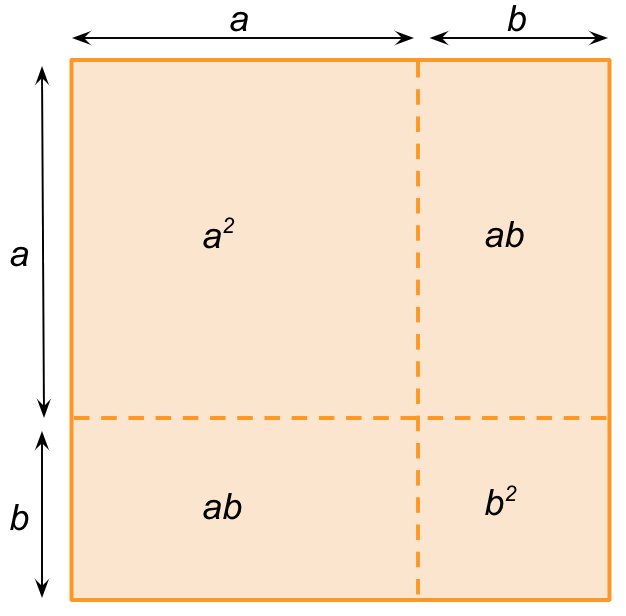So, \begin{align} (a + b)^2 \end{align} is simply the area of this square. Adding the 4 parts we get:

\begin{align} (a + b)^2 = a^2 + ab + ab + b^2 \end{align}

\begin{align} (a + b)^2 = a^2 + 2ab + b^2 \end{align}

### Visualise \begin{align} (a - b)^2 \end{align}

Once again, let’s think of \begin{align} (a - b)^2 \end{align} as the area of a square with length \begin{align} (a - b) \end{align}.
For this, we start with a large square which is $$a^2$$.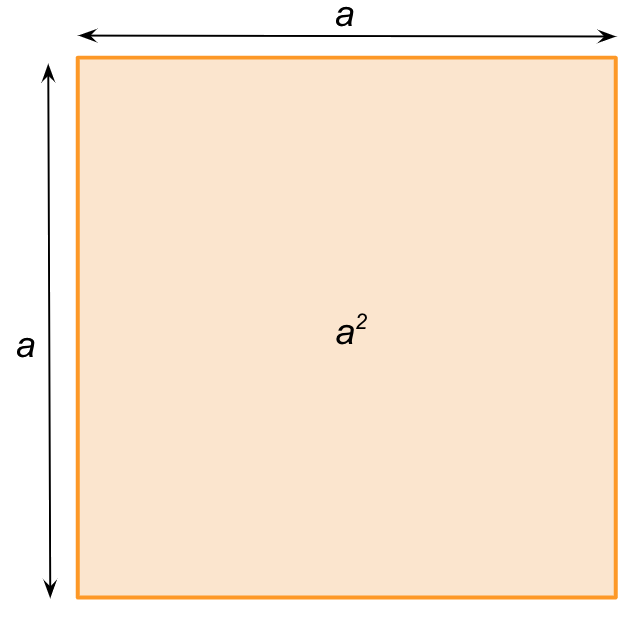We reduce the length of all sides by $$b$$.
So we now have to remove the extra bits from $$a^2$$ to be left with \begin{align} (a - b)^2 \end{align}.
\begin{align} (a - b)^2 \end{align} is shown by the blue area.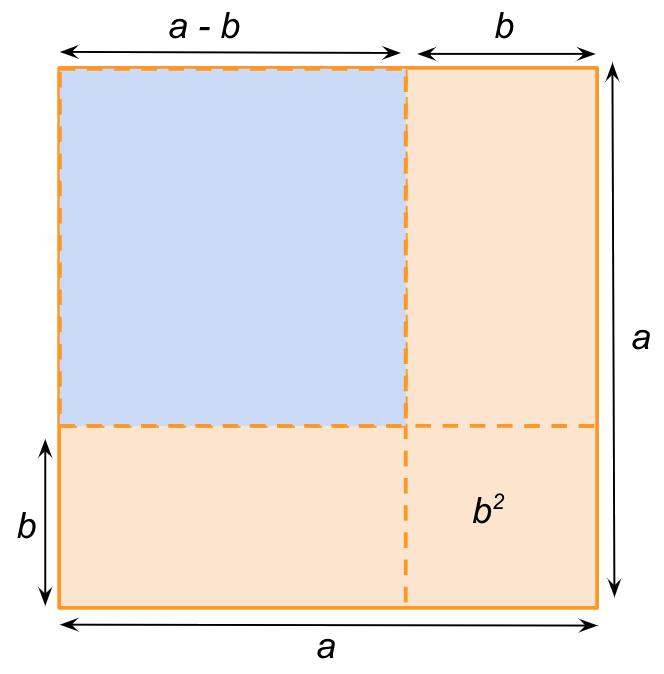To get to this blue area from the larger orange square we have to subtract the vertical and horizontal strips that have the area $$ab$$.

However, removing ab twice ends up removing the overlapping bottom right corner twice. Hence we add $$b^2$$.

\begin{align} (a − b)^2 = a^2 − ab − ab + b^2 \end{align}

\begin{align} (a − b)^2 = a^2 − 2ab + b^2 \end{align}

### Visualise \begin{align} (a + b) (a − b) \end{align}

To visualise this identity, we start once again by visualising a rectangle.

The rectangle will have a length \begin{align} (a + b) \end{align} and breadth \begin{align} (a - b) \end{align}.

The rectangle is shown by the blue and yellow areas together.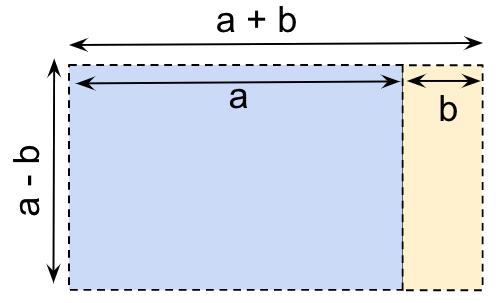Now visualise the yellow vertical strip moving to the bottom of the blue area and aligning horizontally as shown below.

Since we have simply moved it without stretching it, the area remains unchanged.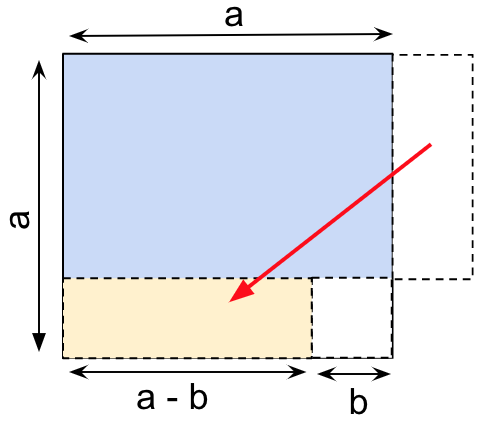When viewed this way, we see that the area is now equal to \begin{align} a^2 - b^2 \end{align}.
\begin{align} (a + b) (a − b) = a^2 - b^2 \end{align}

## Tips and Tricks related to Algebraic Identities

• Visualise identities as rectangles. That way even if you forget, you can quickly work out the expressions.
E.g. \begin{align} (a + b)^2 \end{align} can be visualised as a square with sides\begin{align} (a + b) \end{align} giving four parts: \begin{align} a^2,\;b^2 \end{align} and two $$ab$$.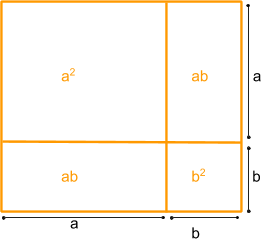• Remember the factored forms rather than the simplified form. It’s difficult to remember what \begin{align} a^2 - b^2 \end{align} factorises to. But if you have the factored form \begin{align} (a + b) (a - b) \end{align}, you can work out the expression even if you don’t remember it.